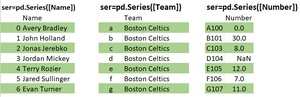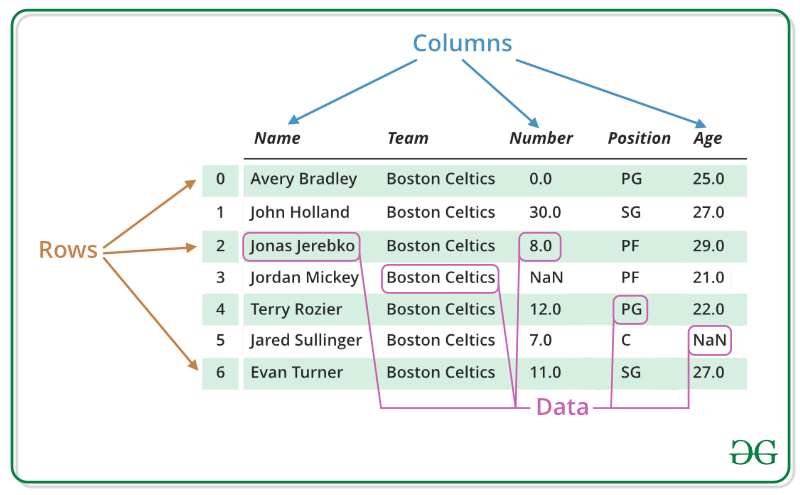GFG App
Open AppBrowser
Continue

# Introduction to Pandas in Python

Pandas is an open-source library that is made mainly for working with relational or labeled data both easily and intuitively. It provides various data structures and operations for manipulating numerical data and time series. This library is built on top of the NumPy library. Pandas is fast and it has high performance & productivity for users.

Table of Content :

History: Pandas were initially developed by Wes McKinney in 2008 while he was working at AQR Capital Management. He convinced the AQR to allow him to open source the Pandas. Another AQR employee, Chang She, joined as the second major contributor to the library in 2012. Over time many versions of pandas have been released. The latest version of the pandas is 1.5.3, released on Jan 18, 2023.

• Fast and efficient for manipulating and analyzing data.
• Data from different file objects can be loaded.
• Easy handling of missing data (represented as NaN) in floating point as well as non-floating point data
• Size mutability: columns can be inserted and deleted from DataFrame and higher dimensional objects
• Data set merging and joining.
• Flexible reshaping and pivoting of data sets
• Provides time-series functionality.
• Powerful group by functionality for performing split-apply-combine operations on data sets.

### Getting Started

The first step of working in pandas is to ensure whether it is installed in the Python folder or not.  If not then we need to install it in our system using pip command. Type cmd command in the search box and locate the folder using cd command where python-pip file has been installed.  After locating it, type the command:

`pip install pandas`

After the pandas have been installed into the system, you need to import the library. This module is generally imported as:

`import pandas as pd`

Here, pd is referred to as an alias to the Pandas. However, it is not necessary to import the library using the alias, it just helps in writing less amount code every time a method or property is called.

Pandas generally provide two data structures for manipulating data, They are:

• Series
• DataFrame

Series: Pandas Series is a one-dimensional labeled array capable of holding data of any type (integer, string, float, python objects, etc.). The axis labels are collectively called indexes. Pandas Series is nothing but a column in an excel sheet. Labels need not be unique but must be a hashable type. The object supports both integer and label-based indexing and provides a host of methods for performing operations involving the index.### Creating a Series

In the real world, a Pandas Series will be created by loading the datasets from existing storage, storage can be SQL Database, CSV file, an Excel file. Pandas Series can be created from the lists, dictionary, and from a scalar value etc.

Example:

## Python3

 `import` `pandas as pd ` `import` `numpy as np`     `# Creating empty series ` `ser ``=` `pd.Series() ` `  `  `print``(ser) `   `# simple array ` `data ``=` `np.array([``'g'``, ``'e'``, ``'e'``, ``'k'``, ``'s'``]) ` `  `  `ser ``=` `pd.Series(data) ` `print``(ser)`

Output:

```Series([], dtype: float64)
0    g
1    e
2    e
3    k
4    s
dtype: object```

### DataFrame

Pandas DataFrame is a two-dimensional size-mutable, potentially heterogeneous tabular data structure with labeled axes (rows and columns). A Data frame is a two-dimensional data structure, i.e., data is aligned in a tabular fashion in rows and columns. Pandas DataFrame consists of three principal components, the data, rows, and columns.### Creating a DataFrame:

In the real world, a Pandas DataFrame will be created by loading the datasets from existing storage, storage can be SQL Database, CSV file, an Excel file. Pandas DataFrame can be created from the lists, dictionary, and from a list of dictionaries, etc.

Example:

## Python3

 `import` `pandas as pd ` `  `  `# Calling DataFrame constructor ` `df ``=` `pd.DataFrame() ` `print``(df)`   `# list of strings ` `lst ``=` `[``'Geeks'``, ``'For'``, ``'Geeks'``, ``'is'``,  ` `            ``'portal'``, ``'for'``, ``'Geeks'``] ` `  `  `# Calling DataFrame constructor on list ` `df ``=` `pd.DataFrame(lst) ` `print``(df) `

Output:

```Empty DataFrame
Columns: []
Index: []
0
0   Geeks
1     For
2   Geeks
3      is
4  portal
5     for
6   Geeks```